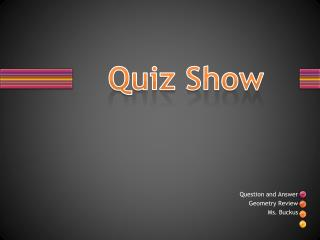# Quiz Show - PowerPoint PPT PresentationDownload PresentationQuiz Show

Quiz ShowDownload Presentation## Quiz Show

- - - - - - - - - - - - - - - - - - - - - - - - - - - E N D - - - - - - - - - - - - - - - - - - - - - - - - - - -
##### Presentation Transcript

1. Quiz Show Question and Answer Geometry Review Ms. Buckus

2. How many faces does a triangular prism have? How many edges? How many vertices? Faces Edges Vertices 5 9 6

3. The point where a polygon sides meet is the vertex

4. How many faces does a rectangular prism have? How many edges? How many vertices? Faces Edges Vertices 6 12 8

5. If a polygon has 27 sides, how many vertices does it have” 27 Examples of Polygons: Triangles, Quadrilateral, Pentagons, Hexagon, Octagons A polygon is a closed plane figure made up of line segment

6. What is right angle? All of the above An angle that is greater than 90 degrees. An angle that forms a straight line. An angle that is less than 90 degrees. An angle that is a square corner. C

7. Draw an example of each polygon. How many sides and vertices does each one have? 4:4 • Quadrilateral • Pentagon • Triangle • Octagon 5:5 3:3 8:8

8. Match the geometric figure to its meaning then draw the figure: 2.line 3.Line Segment 4.Ray 5.Parallel lines A. Never intersecting B. Is a part of a line. It has two end points C. Is an exact location D. Is a path of points that goes on for ever 1.Point E. Is a part of a line. With one end point and one end that goes on forever.

9. Classify each triangle by its sides and then by its angles. 1 Isosceles; acute Scalene; obtuse Scalene; right Equilateral; acute 2 3 4

10. What is the best geometric terms to describe these two butterflies appear to be? Similar& Turned Congruent & Turned Congruent & Flipped Similar & Flipped Congruent & Slide

11. Match the geometric term to the picture. 1. Radius 2. Circle 3.Chord 4.Diameter 5. Center A. B. C. D. E>

12. Use the figure at the right and name:1. An acute angle2.An obtuse angle3.Name a ray4.Name a pair of intersecting lines GPL or KPH GPK or LPH PK, PH, PG, PL GH & LK

13. Do the figures appear to be similar? If so are they congruent? Figure 1-Yes;No Figure 2-Yes; No Figure 3-Yes; No Figure 4-Yes; Yes

14. How many lines of symmetry does each figure have? 1. 6 4 2 2. 3.

15. What is the perimeter of this figure? Unable to get perimeter for this figure 18 sq. cm 33 Sq. cm 18 18 cm

16. The expression for an area of figure is Length plus Width( L + W = Units squared)

17. Find the area of this figure. 21 Sq. Feet

18. Match the geometric figure to its meaning then draw the figure. 1.Intersecting lines 2. Perpendicular lines 3. Equilateral Triangle 4. Isosceles Triangle 5. Scalene Triangle A. No side are the same length. B. All side are the same length. C. Lines that pass through the same point. D. Lines that form right angles. E. At least two sides are the same length.

19. Which polygon has 5 vertices None of these above Pentagon Triangle Quadrilateral Hexagon

20. The Volume of these figure are? Figure 1 is 72 cubic units Figure 2 is 60 cubic units Figure 3 is 60 cubic units Figure 4 is 32 cubic units